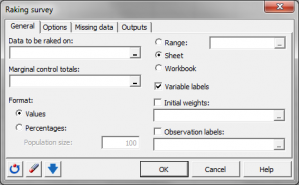# Raking survey data

Raking survey data allocates different weights to respondents so that the sample gets more similar to the population. Available in Excel with XLSTAT.## Principle of raking survey data

When working on surveys, on might need to rake the surveyed sample. That often means looking for raking weights. Sometime, a survey sample may cover a segment of the population with different proportions than in the population itself. The basic idea is to find weights applied to the observations that will give the same proportions in the survey sample as in the population. To do that, we need to use auxiliary variables that are measured both on the survey sample and on the population.

## XLSTAT survey data raking tools

Four methods are available in XLSTAT:

• The raking ratio method It has been developped by Deming and Stefan in 1940.
• The logit method It is close to the raking ratio but lower and upper bounds for the weights are used. These bounds have to be specified by the user.
• The linear method
• The truncated linear method It is the linear method with bounds for the final weights.

## Weight calculation for raking the survey

The weights are calculated using an algorithm called the generalized raking procedure developed by Deville, Särndall and Sautory (1993). The difference from one method to another is the function to be optimized.### analyze your data with xlstat

14-day free trial

Included in

Related features